# Calculating Standard Error of a Sample Mean

##### Rating:
(2)
• (0)
• (0)
• (1)
• (0)
• (1)
Author: Sophia Tutorial
##### Description:

Calculate the standard error of a sample mean.

(more)
Tutorial
what's covered
This tutorial will explain how to calculate standard error for both sample means and sample proportions. It will cover both when the population standard deviation is unknown and known. Our discussion breaks down as follows:

1. Standard Error for Sample Means
2. Standard Error for Sample Proportions (Population Standard Deviation Is Unknown)
3. Standard Error for Sample Proportions (Population Standard Deviation Is Known)

## 1. Standard Error for Sample Means

The standard error for sample means is used when we have quantitative data and can be calculated using the following formula:

formula
Standard Error of a Sample Mean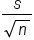It is the sample standard deviation, s, over the square root of the sample size, n.

EXAMPLE

The amount of fallen snow, in inches, is recorded for one week in Minneapolis.

 Day Snow Sunday Monday Tuesday Wednesday Thursday Friday Saturday 1.5 3 4.75 8 0.3 2 2.95

What is the standard error of the sample mean?
The very first question we always want to ask ourselves is, "What type of data are we dealing with? Is this quantitative or qualitative data (which is also known as categorical data)?"
Inches of snow is a quantitative variable, so we're looking for the standard error of a sample mean.
Recall that "s" stands for the standard deviation of the sample, and "n" is the sample size. In this case, we have seven pieces of data for the week, so our sample size is seven.
One way to find the standard deviation is on a graphing calculator. We'll want to enter the data into a list. To get there, go ahead and hit the "Stat" button, and hit "Enter" for edit, and we're going to insert the data into List One.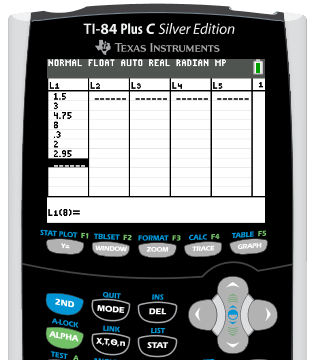At first, we have 1.5 inches of snow, so type 1.5, then hit Enter. Next it snowed 3 inches, then it snowed a lot, 4.75 inches up to 8 inches. Then it tapered off to 0.3 inches. On Friday, there were 2 inches of snow. Finally, on Saturday, there were 2.95 inches of snow.
Once we've entered all of that data, we can exit out of this screen by hitting 2nd Mode. Now, to get s, the standard deviation of this sample, we need to get the sample statistics from that list of data. To do so, hit the Stat button, scroll over to Calc, and we're interested in the first function, one variant statistics.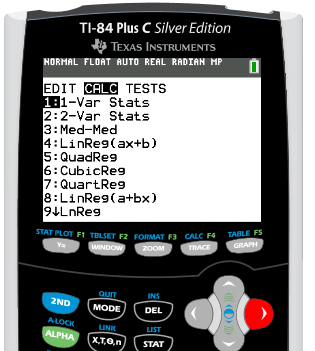Go ahead and hit Enter. We want it for List One, so we're going to hit 2nd 1, and we can see the L1 in the upper left hand corner above the one button. Hit Enter, and we get all sorts of useful data for this set of data.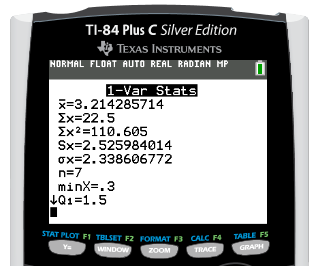We have the x-bar, which is the mean. We also have s of x and sigma of x, as well as the sample size, which is seven.
We also get the five number summary, which is quite useful for other types of problems. However, for this problem, we're interested in the standard deviation of the sample. Remember, this is a sample, not a population, so we want to use the value for s of x, not sigma of x. Remember, population statistics are noted with Greek letters.
In this case, since it is just a sample, we're going to use the 2.526 (which is rounded). We'll divide 2.526 by the square root of 7 to calculate the standard error.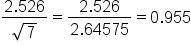The standard error equals 0.955.
Another method to calculate the standard error of the sample mean is in Excel. The first thing we need to do is calculate s, which is the standard deviation from your sample. We'll go to the "Formulas' tab, select the "Statistical" option and look for the standard deviation of a sample. This is indicated with "STDEV.S". The "STDEV.P" is the standard deviation of the population, but remember, we are just dealing with a sample.
Next, insert the data, separating each data value with a comma, and hit Enter.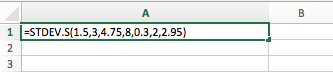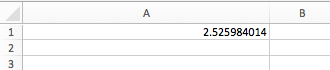Notice how we get the same value that we got in our calculator under s of x. Now, we're going to finish calculating the standard error, which was s divided by the square root of n. In a new cell, we'll type the equal sign and then click on A1 with our mouse since that is where the s-value is. This automatically inserts that value. Next, divide by the square root of n. To get the square root, we have to insert a formula. The square root is just under "Math and Trigonometry", and is indicated with SQRT. Enter the sample size of 7.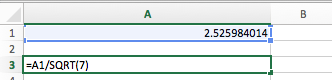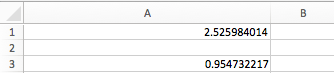Notice how we get the same value as we did on our calculator for standard error, 0.955.

## 2. Standard Error for Sample Proportions (Population Standard Deviation Is Unknown)

Sample proportions use data that is qualitative, or categorical. When calculating the standard error for sample proportions, you need to consider whether or not you know the population standard deviation. If the population standard deviation is NOT known, you will use the following formula:

formula
Standard Error for Sample Proportions (Population Standard Deviation Unknown)The formula to calculate the standard error is p-hat times q-hat, divided by n, all under the square root. We're actually going to use the data that was given to us, which are estimates -- that's what the hat indicates.

EXAMPLE

A survey is conducted at the local high schools to find out about underage drinking. Of the 523 students who replied to the survey, 188 replied that they have consumed some amount of alcohol.

What is the standard error of the sample proportion?

These students are either answering yes or no on the survey: "Yes, I've drank some amount of alcohol" or "No, I have not drank some amount of alcohol". That is qualitative data, also known as categorical data. Therefore, we're dealing with a sample proportion.
Whenever we're dealing with a sample proportion, the next question we need to ask ourselves is, "Do I know the population standard deviation?" In this case, we do not have any of that information. So we will use the standard error formula with the sample data, p-hat and q-hat.
The first thing we need to do is to figure out what p-hat is, based off of the information given to us. In this case, the p-hat is what we're interested in, and that is how many have answered yes to participating in underage drinking. That would be 188 out of 523 students, or 188/523, which is about 36% of the students.
Now, we also need the complement, which would be q-hat. This is also written as 1 minus p-hat. One minus the 188 out of 523, or 1 - 0.36, tells us that 0.64, or 64%, of the students have not participated in underage drinking. To always make sure our math is correct, remember that our p-hat and q-hat should add up to 1, because they're complements of each other.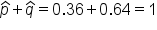Now, we can plug in those values into the formula.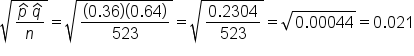We have 0.36 for p-hat, 0.64 for q-hat, and the total sample, n, was 523 students. This calculates to a standard error that is 0.021.

## 3. Standard Error for Sample Proportions (Population Standard Deviation Is Known)

When we have a sample proportion where we do know the population standard deviation, we can use the following formula:

formula
Standard Error for Sample Proportions (Population Standard Deviation Is Known)We do not need to use the sample data, p-hat, to make the estimate for the standard error. We actually know the population parameters we can use this information.

EXAMPLE

Revisiting our prior example, a survey is conducted at the local high schools to find out about underage drinking. Of the 523 students who replied to the survey, 188 replied that they have drank some amount of alcohol. The proportion of underage drinkers nationally is 39%.

What is the standard error of the sample proportion?

We are still looking at the students who were surveyed about underage drinking, but notice how this scenario added on that the proportion of underage drinkers nationally is 39%. We're still calculating the standard error of the sample proportion, but in this case, we know the population standard deviation, which is 39%. We're going to use the formula of square root of pq over n.
We're going to use 39%, or 0.39, for p. This is another way of indicating population proportion. We can then use this to find q, which is the complement of p. The complement of 0.39 is calculated by 1 minus 0.39, which equals 0.61, or 61%. Sometimes we'll see this written as p0 and q0.
The sample size, n, is still 523 students who were surveyed.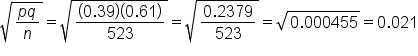The standard error is 0.021.

summary
Today we learned how to calculate standard error, and practiced identifying which formula to use, based on the information given for these three formulas: Sample Means, Sample Proportion (population standard deviation is unknown), and Sample Proportion (population standard deviation is known).

Source: Adapted from Sophia tutorial by RACHEL ORR-DEPNER.

Terms to Know
Standard Error

The standard deviation of the sampling distribution of sample means distribution.

Formulas to Know
Standard Error

Sample Means:Sample Proportion (population standard deviation is unknown):Sample Proportion (population standard deviation is known):Rating Header